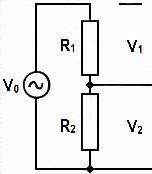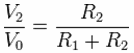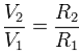Deutsche VersionCalculations:  voltage divider or potentiometer
Voltage drop at the voltage divider

Rule of thumb: The voltages are proportional to the resistances.Formulas:This is an unloaded voltage divider with the four values of the input voltage V0, the output voltage V2, and the divider resistors R1 and R2. Any three values can be entered into the calculator. The fourth value will then be calculated.

 Input voltage V0 volts Upper resistance  R1 ohms Lower resistance R2 ohms Output voltage V2 volts

Voltage division ratio α = Ratio (Output Voltage to Input Voltage) = Vout / Vin
 Z2 = (α × Z1) / (1 − α) dB (level) = 20 × log α Vout = Vin × [Z2 / (Z1 + Z2)]

 Voltage damping D = 20 × log10 ( Vout ) dB ------ ------------- Vin